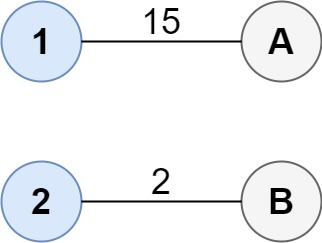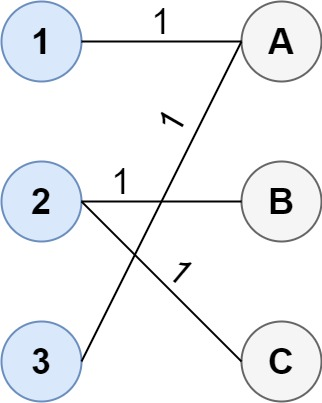# 连通两组点的最小成本

## 1595. 连通两组点的最小成本 (Hard)```输入：cost = [[15, 96], [36, 2]]

1--A
2--B

``````输入：cost = [[1, 3, 5], [4, 1, 1], [1, 5, 3]]

1--A
2--B
2--C
3--A

```输入：cost = [[2, 5, 1], [3, 4, 7], [8, 1, 2], [6, 2, 4], [3, 8, 8]]

```

• `size1 == cost.length`
• `size2 == cost[i].length`
• `1 <= size1, size2 <= 12`
• `size1 >= size2`
• `0 <= cost[i][j] <= 100`

### 相关话题

[位运算] [数组] [动态规划] [状态压缩] [矩阵]